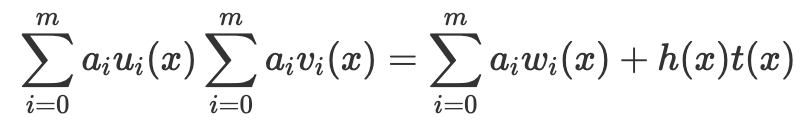top of page
• Jeffrey Scholz

# Encrypted Evaluation of a Quadratic Arithmetic Program

Updated: 2 days agowhich was derived from an Rank 1 Constraint System (R1CS) of the form Ls ⊙ Rs = Os

where uᵢ(x), vᵢ(x), wᵢ(x) are the polynomial interpolations (indexed on the original column) of the R1CS matrices L, R, and O corresponding to the left wires, the right wires, and the output wires of the arithmetic circuit. (a₀,…,aₘ) is the witness vector where a₀ is hardcoded to 1. The vectors s and a hold the same values.

Specifically, u₁(x) is the polynomial obtained from the interpolation of the first column of L, u₂(x) is the interpolation of the second column, and so forth. The same applies for R and O but with v and w respectively.

## A concrete example

To make this a little less abstract, let’s say that matrix L, R, and O have 4 rows and 3 columns.

Since we have 4 rows, it means our interpolating polynomials will be of degree 3. Because we have 3 columns, each matrix will result in 3 polynomials (for a total of 9).

After we transform them to a QAP, we will obtain the coefficients of the polynomials as follows:Here, we mean our notation u₂₃ means the coefficient of the second polynomial (i.e. interpolated from the second column) of u associated with the x³ term of that polynomial.

So saying u₂(x) is equivalent toThe prover wishes to evaluate the QAP at point τ, i.e.where τ is chosen randomly at the trusted setup, thus allowing the verifier to test polynomial equality at that point.

## Trusted Setup

To do an encrypted evaluation of the polynomial, we need a trusted setup.

The agent doing the trusted setup picks a random value τ and for the generators G1 and G2 createsIn our notation, a variable surrounded by a square bracket is an elliptic curve point. The subscript 1 or 2 that follows indicates which elliptic curve group it belongs to.

Although the original value that generated the elliptic curve point is visible in our notation, the prover and the verifier cannot extract it due to the discrete logarithm problem.

## Evaluation of uᵢ(τ), vᵢ(τ), wᵢ(τ)

The prover can evaluate the nine polynomials uᵢ(τ), vᵢ(τ), wᵢ(τ) by taking the inner product of the elliptic curve points with the coefficients of the polynomial. Since we are doing an encrypted evaulation, we naturally get an encrypted output, i.e. an elliptic curve point.

For example,Now that we know how to evaluate uᵢ(τ), vᵢ(τ), wᵢ(τ), then computing:is straightforward. We just need to evaluate the final term in an encrypted manner.

## Evaluating h(τ)t(τ)

The polynomial t(x) is determined by the circuit. In our case, since we have n = 3 rows, t(x) = (x - 1)(x - 2)(x - 3).

The polynomial h(x) is computed by the prover viaNote that this is a symbolic calculation, not an encrypted evaluation. The prover actually must expand and multiply and divide all the polynomials at play here. This is a very expensive operation, one of the primary reason zk-snarks take a lot of work to prove.

The division will have no remainder if and only if the polynomials in the numerator truly interpolates (1,0), (2,0), (3,0). This is homomorphic to our R1CS having a valid solution, i.e.

Ls ⊙ Rs - Os = 0.

### We don’t want to multiply the encrypted evaluation of h and t

Because an encrypted evaluation results in an elliptic curve point, this would force us to introduce a pairing to evaluate the multiplication of h(τ) and t(τ). To avoid this, we do something more clever in the trusted setup phase.

Because t(x) is known at the setup phase, and we know it has a power of 3 (it’s part of the definition of the circuit), the setup agent can do the following:We can simply take the inner product of the coefficients of h(x) with the trusted setup elements above to do an encrypted evaluation of h(τ)t(τ).This might seem like an unusual way to multiply polynomials, but observe that the following three operations are equivalent if we have a fixed evaluation point:

• evaluating t at τ then multiplying t(τ) by h then evaluating that at τ

• multiplying h by t symbolically, then evaluating it at τ

• evaluating h(τ) and t(τ) separately, then multiplying the results

In other words h(τ)t(τ) = ht(τ) = (h(x) * t(τ))(τ)

If this still feels a little strange, consider an example in code form:

```from numpy import poly1d
h = poly1d([1, -10])*poly1d([2, 5])
t = poly1d([1, -1])*poly1d([1, -2])*poly1d([1, -3])

tau = 14
# our algorithm evaluates using the method of the last element, but in encrypted form
assert (h*t)(tau) == h(tau)*t(tau) == poly1d(t(tau) * h)(tau)```

## The final evaluation

Given a circuit transformed to a QAP defined with polynomials u(x), v(x), w(x) and polynomial t(x), and given a trusted setupWe compute three elliptic curve points [A]₁, [B]₂, [C]₁ such thatAnd the verifier will compute

`pairing([A]₁,[B]₂) == pairing([C]₁,[G₂]₂)`

and accept if the equality is true.

This is homomorphic to a verifier using an encrypted witness for an R1CS.

On the right hand side, we are effectively “multiplying by one” when we multiply by the generator of G2. This is to make both sides G12 points, and thus comparable.

At this point, we have a fully functional and succinct zero knowledge proof but we have the obvious problem that we have no idea if the prover really derived [A]₁, [B]₂, [C]₁ from the trusted setup using the QAP or simply invented them. We also don’t have a way of incorporating public inputs. All we have is a proof the prover has a valid witness with none of the elements being public.

All of this will be addressed in our tutorial for the groth16 algorithm.